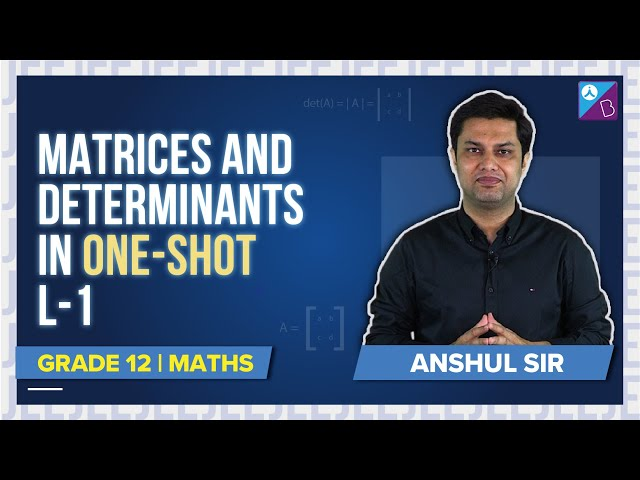Checkout JEE MAINS 2022 Question Paper Analysis : Checkout JEE MAINS 2022 Question Paper Analysis :

# Determinants For Class 12

Determinants for Class 12 notes are available here, as per the syllabus guided by the boards. We have already learned that the algebraic equations can be expressed in the form of matrices. To determine the uniqueness of solution associated with such matrices, we find the determinant. It has huge applications in Engineering, Economics, Science, etc. Here we will learn the determinants up to order 3 with real entries. Apart from it, determinants for class 12 covers the matrices properties, cofactors and applications of matrices and determinants along with finding the area of a triangle.

## Determinants for class 12 Topics

The topics covered in determinants for class 12 includes:

• Introduction
• Determinant Definition
• Properties
• Area of a Triangle
• Minors and Cofactors
• Adjoint and the inverse of a Matrix
• Determinants and Matrix Applications

## Determinants for Class 12 Notes

Definition: Every square matrix A of the order n, can associate a number called determinants of the square matrix A.

Determinant of the order one (1×1)

Consider a matrix A = [a], then the determinant of the matrix is equal to a.

Determinant of the order Two (2×2)

If the order of the matrix is 2, then the determinants is defined matrix A, where A is-

$$\begin{array}{l}A= \begin{bmatrix} a_{11} & a_{12}\\ a_{21} & a_{22} \end{bmatrix}\end{array}$$

Determinant of A =

$$\begin{array}{l}\left |A \right |= \begin{vmatrix} a_{11} & a_{12}\\ a_{21} & a_{22} \end{vmatrix}\end{array}$$

$$\begin{array}{l}= a_{11}. a_{22} – a_{21}.a_{12}\end{array}$$

Similarly we can find the determinant of the order 3×3

Determinant of the Order Three (3×3)

Suppose a matrix A of order three is given:

$$\begin{array}{l}A= \begin{bmatrix} a_{11} & a_{12} & a_{13}\\ a_{21} & a_{22} & a_{23}\\ a_{31} & a_{32} & a_{33} \end{bmatrix}\end{array}$$

Then the determinant for a 3×3 matrix is given by;

|A| = a11 a22 a33 – a11 a23 a32 – a12 a21 a33 + a12 a23 a31 + a13 a21 a32 – a13 a31 a22

### Properties of Determinants

Let us now look on to the properties of the Determinants which is discussed in determinants for class 12:

Property 1- The value of the determinant remains unchanged if the rows and columns of a determinant are interchanged.

Property 2- If any two rows (or columns) of determinants are interchanged, then sign of determinants changes.

Property 3- If any two rows or columns of a determinant are equal or identical, then the value of the determinant is 0.

Property 4- If each element of a row or a column is multiplied by a constant value k, then the value of the determinant originally obtained is multiplied with k.

### Area of Triangle Using Determinant

We have already learned, that the area of a triangle whose vertices are (x1, y1), (x2, y2) and (x3, y3) is given by;

A = 1/2[x1(y2–y3) + x2(y3–y1) + x3(y1–y2)]

Now, we can write the above expression in the form of the determinant as;

$$\begin{array}{l}\Delta = \frac{1}{2}\begin{vmatrix} x_{1} & y_{1} & 1\\ x_{2} & y_{2} & 1\\ x_{3} & y_{3} & 1 \end{vmatrix}\end{array}$$

### Minors and Cofactors

Minor of an element aij of a determinant is the determinant obtained by deleting its ‘ith’ row and ‘jth’ column in which element aij lies. Minor of an element aij is denoted by Mij. Minor of an element of a  determinant of order n(n ≥ 2) is a determinant of order n – 1. Or in simple words we can say, the minor of any element in a determinant is the determinant of just the single element. Suppose,

$$\begin{array}{l}\Delta = \begin{vmatrix} a & b & c\\ d & e & f\\ g & h & i \end{vmatrix}\end{array}$$
is a determinant and we have to find the minor of element ‘f’, then, we can write;

$$\begin{array}{l}M_{f}=\begin{vmatrix} a & b\\ g & h \end{vmatrix}\end{array}$$

Mf = a.h – b.g

Cofactor of an element aij in a determinant is defined by;

Aij= (-1)i+jMij

Apart from these topics, there are few more topics covered in chapter 4 of class 12 Maths, such as;

• adjoint and inverse of a square matrix
• consistency and inconsistency of linear equations
• the solution of linear equations in two or three variables using the inverse of a matrix

## Video Lesson on Matrices and Determinants Class 12### Determinants for class 12 Examples

Example:

Find the area of a triangle whose vertices are (2,4), (-4,1) and (4,1).

Solution:

By the formula of area of a triangle, written in determinant form, we can write;

$$\begin{array}{l}\Delta = \frac{1}{2}\begin{vmatrix} 2 & 4 & 1\\ -4 & 1 & 1\\ 4 & 1 & 1 \end{vmatrix}\end{array}$$

∆ = 1/2 [2(1-1) – 4(-4-4)+1(-4-4)

∆ = 1/2 [-4(-8) + (-8)]

∆ = 1/2 [ 32 – 8]

∆ = 1/2 x 24

∆ = 12 sq.unit.

Example 2:

Find the minor and cofactor of third element in determinant

$$\begin{array}{l}\begin{vmatrix} 1 & -2\\ 4 & 3 \end{vmatrix}\end{array}$$
.

Solution:

Here the third element is 4.

So, minor of element of 4 = -2

and cofactor of element 4 = (-1)2+1(Minor of element 4)
A21 = (-1)3(-2) = (-1)(-2) = 2

Find the notes and revision materials for all the topics here at BYJU’S- The Learning App by subscribing with us.